# Team:Tsinghua/Model

Neon Coli-Necessary Expression Only

## Model

#### 1.Introduction

1.1 Why do we need a model
Developing a mathematical model of the systems we established (or plan to establish) is a vital step in our project. A model, namely, is a mathematical representation of the system. The model can help us with the following:
- Confirming that our system has the potential to work as expected. For example, as for the Test System, the model should present us that both the self-activating component and safety-catch component work in theory.
- Determining optimal conditions for the system to work. e.g. Initial concentration of AHL.
- Estimating biological parameters.
- Understanding the mechanism of the system better.
- Optimizing the design of our final project.

1.2 What is our model about?
The model is basically established by using ODEs (ordinary differential equations) to describe reactions and events in the system. ODE is a powerful tool when it comes to modelling a dynamic system. It allows us to predict the behavior of the system at given conditions, and to analyze several features of the system such as robustness and stability. For the sake of simplicity, various assumptions are made when developing the model. These assumptions usually tend to simplify our systems, reducing our workload. According to the assumptions, many trivial details are omitted, while the overall properties and behaviors of the model remain unchanged.
When describing the reactions and events involved, we mainly apply the law of mass action, which assumes the system is in quasi steady state. When modelling reactions that resemble enzymatically catalyzed reactions and events that involve ligand binding, we refer to Hill Equation, which is simple but effective.
We focused on building a model for the “Test System”. The model depicts the behaviors of the Test System under several different conditions, and highlights some key features and properties of the system. The model is generally successful as it predicted the behavior of the system correctly, according to our experiments. We have performed various analysis regarding the model and pinpointed some intriguing characteristics of the system.
In addition to this, we also built a model for the Neon System. Using the model, we can simulate how Neon System works. It can serve as a “guideline” for our future work on the Neon System.

#### 2. Model Development —— Test System

2.1 Overview
In the following sections, we will explain how we developed our model of the Test System and present our results. Figure 1 offers an intuitive explanation of the way our Test System works. It can be seen that the system would act differently (perform different functions) under different conditions. Our goal is to specifically describe the system given different conditions, using ODE. The concentrations of the chemical species in the system are regarded as variables, which change over time. Thus, depicting how the concentrations of the molecules of interest change is the primary step.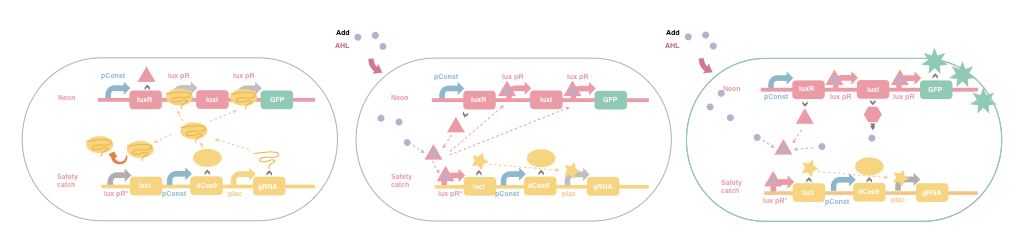Figure 1 Illustration of How the System Works

2.2 Notations of Important Variables and Chemical Species
In the model, a set of variables representing the concentrations of various chemical species in the system are used. All these variables are functions of time. The notations and corresponding meanings are listed below.
$H(t)$——concentration of 3OC6HSL in the cell
$I(t)$——concentration of luxI
$H_{ex}(t)$——concentration of 3OC6HSL outside the cell (in the medium)
$C(t)$——concentration of 3OC6HSL-LuxR complex
$R(t)$——concentration of LuxR monomers
$G(t)$——concentration of GFP
$Cas(t)$——concentration of dCas protein in the cell
$C^*(t)$——concentration of dCas/gRNA complex
$gRNA(t)$——concentration of unbound gRNA
$I^*(t)$——concentration of lacI

2.3 Details of Model Development
Next, we will dissect the reactions and events involved in the model. For each reaction involved, we will present the relevant assumptions, molecules involved, and formulae and parameters related to reaction rate or steady state.

1) AHL synthesis inside the cell
- Reaction: Precursors $\to$ AHL (3OC6HSL)
- Reaction rate: $v=k_0 I(t)$
- Relevant parameters: $k_0 \Rightarrow$ rate of synthesis of 3OC6HSL
Explanation/Assumptions: In bacteria, AHL is synthesized from its precursors via several enzymatic reactions. The last step of synthesis is catalyzed by protein luxI. Due to the scarcity of luxI protein in our system, it can be seen as the rate-limiting factor in the synthesis reaction. Thus, we assume the rate of synthesis of AHL is directly proportional to the concentration of luxI.

2) Diffusion of AHL out of the cell
- Event: AHL (in the cell) $\to$ AHL (outside the cell) - Rate:$v=r(H(t)-H_{ex}(t))$ - Relevant parameter: $r \Rightarrow$ coefficient of diffusion Explanation: In reality, diffusion of AHL across the membrane is a relatively complicated process. If we see the diffusion of AHL across cell membrane as simple diffusion, the process can be described using Fick's Law, which assumes that the flux of molecules across a membrane is proportional to the gradient concentration.
$$J=-D\frac{dc}{dx}$$
Approximately, this means the change of number of molecules on one side of the membrane is proportional to the difference of concentration across the membrane. In our model, we assume that the diffusion of AHL across the membrane is fast enough so that we can omit the process in which AHL travels within the membrane. Also, it is assumed that the distribution of AHL in the medium and inside cells can come to a steady state in a very short time, which means the system is generally homogeneous when it comes to AHL distribution. Previous iGEM teams have discussed the details of AHL diffusion in the extracellular medium, and we believe these details prove to play insignificant roles in our prospect so they are not included in the model.

3) Diffusion of AHL into the cell
- Event: AHL (outside the cell) $\to$ AHL (in the cell)
- Rate:$v=pr(H_{ex}(t)-H(t))$
- Relevant parameters: $p \Rightarrow$ coefficient of population density.
Explanation: This process is the reverse version of the previous one. A coefficient of population density is multiplied to the gradient concentration, as the number of bacteria in the system would affect how quickly the external AHL concentration changes.

4) Formation of 3OC6HSL-LuxR Complex
- Reaction: 2*3OC6HSL + 2 LuxR $\to$ 3OC6HSL-LuxR Complex
- Reaction rate: $v=2k_1(H(t))^2(R(t)-2C(t))^2$
- Parameter: $k_1\Rightarrow$rate constant of complex formation
Explanation: This is based on the law of mass action. Two molecules of 3OC6HSL binds to one luxR protein to form a complex, which can then activate the transcription of luxpR promoters.

5) Dissociation of 3OC6HSL-LuxR Complex
- Reaction: 3OC6HSL-LuxR Complex$\to$2 3OC6HSL + 2 LuxR
- Reaction rate (Re-formation of AHL) : $v=2k_2C(t)$
- Parameter: $k_2\Rightarrow$rate constant of complex dissociation
Explanation: This is based on the law of mass action.

6) Degradation of AHL inside the cell
- Reaction: 3OC6HSL $\to$ NULL
- Reaction rate : $v=d_1H(t)$
- Parameter: $d_1\Rightarrow$rate constant of AHL degradation inside the cell
Explanation: This is based on the law of mass action. We assume that AHL gradually degrades in the cell, while the rate is proportional to its current concentration.

7) Degradation of AHL outside the cell
- Reaction: 3OC6HSL $\to$ NULL
- Reaction rate : $v=d_2H_{ex}(t)$
- Parameter: $d_2\Rightarrow$rate constant of AHL degradation outside the cell
Explanation: This is based on the law of mass action. Similar to the previous one.

8) Binding of lacI to the placI promoter
- Reaction: lacI+placI $\to$ lacI bound to placI
- Proportion of bound sites: $c_{lac}=\frac{I_l(t)}{K_d^*(t)+I^l(t)}$
- Parameter: $K_d^*\Rightarrow$ Binding affinity of lacI to placI

9) Production of guide RNA
- Reaction: NULL $\to$ gRNA
- reaction rate : $v=a_g*(1-c_{lac})$
- Parameter: $a_g\Rightarrow$ Expression rate of gRNA when placI is not repressed
Explanation: Only promoters that are not repressed by lacI can support the transcription of gRNA.
- Reaction: gRNA $\to$ NULL
- reaction rate : $v=d_g*G(t)$
- Parameter: $d_g\Rightarrow$ Rate constant of degradation of gRNA

- Reaction: dCas9 $\to$ NULL
- reaction rate : $v=d_c*Cas(t)$
- Parameter: $d_c\Rightarrow$ Rate constant of degradation of dCas9

12) Production of dCas9 protein
- Reaction: NULL $\to$ dCas9
- reaction rate : $v=a_0$
- Parameter: $a_0\Rightarrow$ Efficiency of the constant promoter

13) Production of dCas9-gRNA complex
- Reaction: dCas9+gRNA $\to$ dCas9-gRNA complex
- reaction rate : $v=k_3gRNA(t)Cas(t)$
- Parameter: $k_3\Rightarrow$ Rate constant of dCas9 complex formation
Explanation: This is based on the law of mass action.

14) Dissociation of dCas9-gRNA complex
- Reaction: dCas9-gRNA complex$\to$dCas9+gRNA
- reaction rate : $v=k_4C^*(t)$
- Parameter: $k_4\Rightarrow$ Rate constant of dCas9 complex dissociation
Explanation: This is based on the law of mass action.

15) Binding of dCas9 complex to the luxPR promoter
- Reaction: dCas9-gRNA complex+luxPR $\to$ inhibited luxPR
- Proportion of bound sites : $c_{crispr}=\frac{C^*(t)}{K_c^*(t)+C^*(t)}$
- Parameter: $K_c^*\Rightarrow$ Binding affinity of dCas9 complex to the luxPR promoter.

16) Production of luxR protein
- Reaction: NULL $\to$ luxR
- reaction rate : $v=a_0$
- Parameter: $a_0\Rightarrow$ Efficiency of the constant promoter

- Reaction: luxR $\to$ NULL
- reaction rate : $v=b_2R(t)$
- Parameter: $b_2\Rightarrow$ Rate constant of degradation of LuxR

18) Binding of 3OC6HSL-LuxR Complex to luxPR promoter
- Reaction: 3OC6HSL-LuxR Complex+ luxPR promoter$\to$ activated luxPR promoter
- Proportion of bound sites: $x=\frac{a_1(C(t))^{\beta_1}}{2(K_m^{\beta_1}+(C(t))^{\beta_1})}$
- Parameter: $K_m\Rightarrow$ Binding affinity of the complex to luxPR promoter
$beta_1\Rightarrow$Hill coefficient for the binding of complex to luxpR
Explanation: This is based on Hill equation.

19) Production of luxI protein
- Reaction: NULL $\to$ luxI
- reaction rate : $v=(1-c_{crispri})*(l_1+a_1*x)$
- Parameter: $l_1\Rightarrow$ Leaky expression efficiency of lux pR promoter
$a_1\Rightarrow$ Enhanced expression efficiency of lux pR when bound to the complex. (The increment)
Explanation: We assume that all the sites bound by the dCas9 complex are completely inactivated, thus they are unable to produce any product.

- Reaction: luxI$\to$ NULL
- reaction rate : $v=b_1*I(t)$
- Parameter: $b_1\Rightarrow$ Rate constant of degradation of luxI

21) Production of GFP
- Reaction: NULL $\to$ GFP
- reaction rate : $v=(1-c_{crispri})*(l_1+a_1*x)$
- Parameter: $l_1\Rightarrow$ Leaky expression efficiency of lux pR promoter
$a_1\Rightarrow$ Enhanced expression efficiency of lux pR when bound to the complex.(The increment)
Explanation: We assume that all the sites bound by the dCas9 complex are completely inactivated, thus they are unable to produce any product.

- Reaction: GFP$\to$ NULL
- reaction rate : $v=b_3*gRNA(t)$
- Parameter: $b_3\Rightarrow$ Rate constant of degradation of GFP

23) Production of lacI
- Reaction: NULL $\to$ lacI
- reaction rate : $v=(l_1+a_1*x)$
- Parameter: $l_1\Rightarrow$ Leaky expression efficiency of lux pR promoter
$a_1\Rightarrow$ Enhanced expression efficiency of lux pR when bound to the complex.(The increment)
Explanation: This lux pR promoter is not inhibited by CRISPRi

- Reaction: lacI$\to$ NULL
- reaction rate : $v=d_l*I^*(t)$
- Parameter: $d_l\Rightarrow$ Rate constant of degradation of lacI

25) Binding of IPTG to lacI
- Reaction: lacI+IPTG $\to$ inactivated lacI
- Property: Since lacI a homotetramer, IPTG can bind to any of the four subunits t inactivate it.
- Concentration of active lacI (presumably at steady state): $I_l(t)=I^*(t)(\frac{K_i}{K_i+IPTG})^4$
- Parameter: $K_i \Rightarrow$ Dissociation constant of lacI-IPTG
Based on all the reactions and events listed above, we are then able to explicitly describe how the concentration of each type of molecules of interest change over time. We will integrate the information we have so far and begin to write out the equations.
The first ODE describes the change of AHL concentration inside a cell. The time scale taken into consideration is relatively short, so we omit the impact of cell division and death. The change of AHL concentrations involves AHL synthesis, degradation, diffusion and binding to LuxR.
$$\frac{d}{dt}H(t)=k_0I(t)-r(H(t)-H_{ex}(t))-2k_1(H(t))^2(R(t)-2C(t))^2+2k_2C(t)-d_1H(t)$$ The following equation describes how the concentration of luxR-AHL complex changes over time. $$\frac{d}{dt}C(t)=k_1(H(t))^2(R(t)-2C(t))^2-k_2C(t)$$ The following equations define the inhibitory effects of CRISPRi and lacI protein on their target sequences, respectively. $$c_{crispri}=\frac{C^*(t)}{K_m^*(t)+C^*(t)}$$ $$c_{lac}=\frac{I_l(t)}{K_d^*(t)+I_l(t)}$$ LuxI protein concentration is supposed to change the way described by the ODE below. $$\frac{d}{dt}I(t)=(1-c_{crispri})*[l_1+\frac{a_1(C(t))^{\beta_1}}{(K_m^{\beta_1}+(C(t))^{\beta_1})}]-b_1I(t)$$ The dynamics of luxR monomer is rather simple, as described below. $$\frac{d}{dt}R(t)=a_0-b_2R(t)$$ The change of concentration of AHL outside the cell mainly depends on dilution effect and cross-membrane diffusion. $$\frac{d}{dt}H_{ex}(t)=pr(H(t)-H_{ex}(t))-d_2H_{ex}(t)$$ The following equation describes how GFP is produced under regulation. $$\frac{d}{dt}G(t)=(1-c_{crispri})*[\frac{a_1(C(t))^{\beta_1}}{(K_m^{\beta_1}+(C(t))^{\beta_1})}+l_1]-b_3G(t)$$ And finally, the equations below describe the dynamics of CRISPRi system. $$\frac{d gRNA(t)}{dt}=a_g*(1-c_{lac})-k_3gRNA(t)Cas(t)+k_4C^*(t)-d_ggRNA(t)$$ $$\frac{dC^*(t)}{dt}=k_3gRNA(t)Cas(t)-k_4C^*(t)$$ $$\frac{dCas(t)}{dt}=a_0-k_3gRNA(t)Cas(t)+k_4C^*(t)-d_cCas(t)$$ $$\frac{dI^*(t)}{dt}=l_1+\frac{a_1(C(t))^{\beta_1}}{(K_m^{\beta_1}+(C(t))^{\beta_1})}-d_l*I^*(t)$$ With these equations, we will soon be able to run a simulation of the system. Before we obtain the result of the simulations, we are required to set the values of biological parameters and set the initial state of the system. That, is another story…

2.4 Setting the Parameters and Initial States
How do we acquire the parameters we need to simulate and validate our model? Estimating all the parameters based on our experimental results is not realistic, due to the large number of parameters, the nonlinearity of the system and the potential inaccuracy of our experiments. A straightforward way to obtain the values of parameters is to refer to literatures, results of previous iGEM teams and common sense. We trust that the simulations run based on these values of parameters should provide us with generally satisfying results, which can at least predict the general behavior of the system correctly.
The values of parameters we utilize are listed below.

Table 1 Biological Parameters Used in the Model

Name Description Value Unit Source
k0 rate of synthesis of 3OC6HSL 0.04 min-1 Ref
r coefficient of diffusion 100 min-1 Ref
k1 rate constant of complex formation 0.1 nM-1min-1 Ref
k2 rate constant of complex dissociation 10 min-1 Ref
d1 rate constant of AHL defradation inside the cell 0.004 min-1 Estimated by ourselves
d2 rate constant of AHL degradation outside the cell 0.0004 min-1 Estimated by ourselves
Kd Binding affinity of lacI to placI 10.49 nM PAMDB database
ag Transcription rate of placI (not repressed) 5 nMmin-1 Ref
dg Rate constant of degradation of gRNA 0.42 nM min-1 Ref
dc Rate constant of degradation of dCas9 0.14 nMmin-1 Ref
a0 Efficiency of the constant promoter 1 nM min-1 Estimated by ourselves
k3 Rate constant of dCas9 complex formation 0.0108 nM-1min-1 Ref
k4 Rate constant of dCas9 complex dissociation 0.00001 min-1 Ref
Kc Binding affinity of dCas9 complex to target sequence 0.025 nM Ref
b2 Rate constant of degradation of LuxR 0.0231 min-1 Team:ETH_Zurich 2014
Km Binding affinity of the complex to lux pR promoter 10 nM Team:ETH_Zurich 2014
l1 Leaky expression efficiency of lux pR promoter 0.02 nM min-1 Estimated by ourselves
a1 Enhanced expression efficiency of lux pR on the Neon plasmid when bound to the complex.(The increment) 5 nM min-1 Team:Tokyo Tech 2012
a2 Enhanced expression efficiency of lux pR on the Safety Catch plasmid when bound to the complex.(The increment) 5 nM min-1 Team:Tokyo Tech 2012
b1 Rate constant of degradation of luxI 0.0002 min-1 Team:ETH_Zurich 2014
b3 Rate constant of degradation of GFP 0.005 min-1 Estimated ourselves
dl Rate constant of degradation of lacI 0.023 min-1 PAMDB database
The initial concentrations of several species, such as dCas9 and guide RNA, which are fairly difficult to "guess" and nowhere to be found in literatures, can be better estimated by running simplified models only regarding the equilibrium of the relevant molecules. Here, we present an example run by SimBiology App on MATLAB. The diagram of the model is shown below.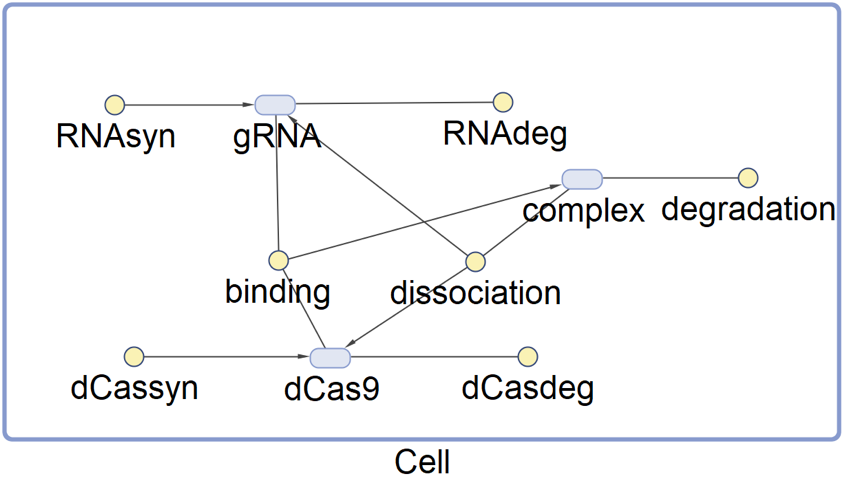Figure 2 Graphical Representation of the SimBiology Model for Determining Initial Concentrations of CRISPRi-Related Species

Running the model with relevant parameters set on SimBiology can provide us with diagrams showing the result of simulation. The result of the simulation provides us with initial concentrations of the relevant molecules which are probably more believable.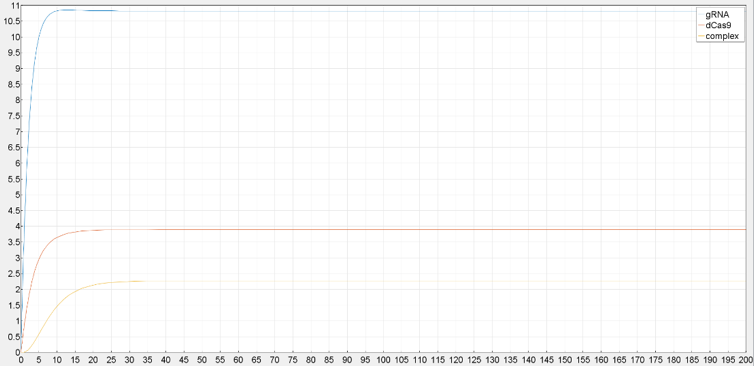Figure 3 Results of SimBiology Model. Simulated Concentrations of CRISPRi-Related Species at Equilibrium are Shown.

#### 3.Model Simulation—— Test System

3.1 Effect of AHL concentration
According to our expectations, the Test System is inducible upon the addition of external AHL. With proper concentrations of AHL, the cells would undergo a series of changes regardin gene expression. The most obvious change is the increase of GFP concentration in the cell, for us. The “safety catch” component also ensures that the system has minimal leakage, meaning that minor fluctuations in the system when no AHL is added, or very trace amount of AHL should not activate the system. To test whether the Test System does possess these features, we will run a series of simulations of our model.
The parameter values used in the model are stated in previous parts. As for the intial states, the concentrations of CRISPRi related molecules are set according to the SimBiology simulation results. Concentrations of most molecules that are not supposed to be synthesized without AHL are set to 0. External AHL concentrations are tuned to reveal the effect of AHL concentration on the system.
To begin with, we set intial external AHL concentration as 10-6 M, which is relatively high. The simulation result is shown below.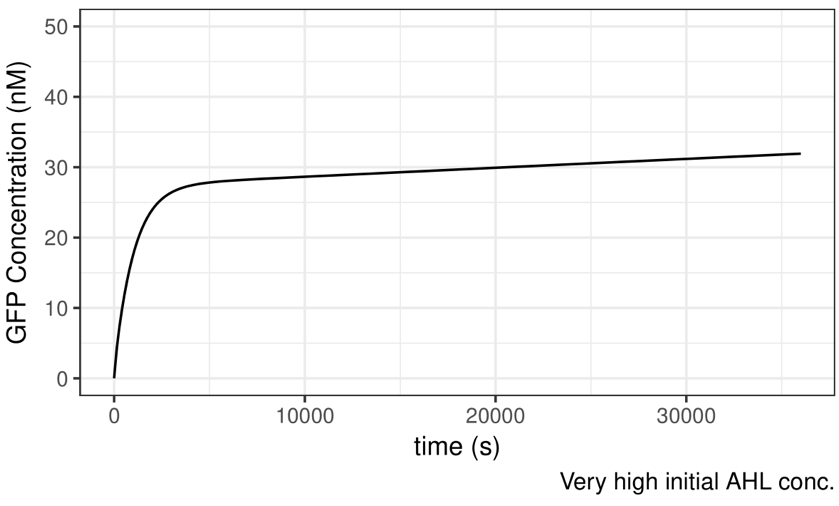Figure 4 Simulation Result——With Very High Initial AHL Concentration

The result meets our expectation. According to the plot, GFP concentration will begin to rise exponentially following the addition of AHL. The velocity of increment decreases gradually, till approximate saturation is reached. The simulation result implies that the Test System is inducible.
What happens when lower concentrations of AHL is added? The following plots represent 10-7 and 10-9 M of AHL concentration, respectively.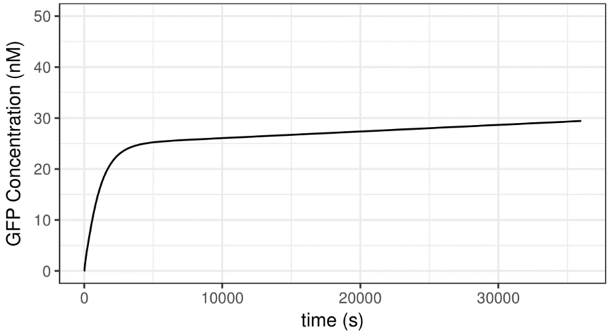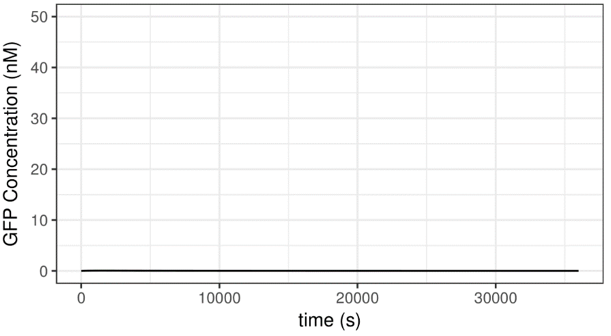Figure 5 Results of Varied AHL Concentrations

As can be deduced from these plots, the decrease of AHL concentration from 10-6 to 10-7 has no substantial influence on the behavior of the system. Through further analysis, we found that the rate of GFP concentration increment is indeed higher when 10-6 M of AHL is added than when 10-7 M of AHL is added, however this difference can merely be detected after 100 seconds. Given these two different initial states, the state of the system will eventually become quite same. For one, this shows that the system is robust, to some extent. In addition to this, this phenomenon makes sense and has biological interpretations. Within a certain range of concentrations, AHL serves as a “trigger” which turns the system on by enabling expression of several proteins, so it has an “all-or-none” effect, to some extent.
When the concentrations of AHL is further decreased, to 10-9 M, for instance, the behavior of the system is altered dramatically. When AHL concentration is this low, the system cannot be activated and no GFP is expressed. This is partial thanks to the “safety catch” design. This result gives us confidence that leakage can be prevented with the presence of minor fluctuations.
You can interact with the plot down below. Use mouse to drag the slider to tune the initial AHL concentration and observe how this will influence GFP production in the cells!
Summary:
- Increased AHL concentration tends to result in faster GFP production.
- A threshold for AHL concentration exists. AHL concentration below this threshold cannot activate the system thanks to the safety catch mechanism.

3.2 Effect of lux pR efficiency
Lux pR, a promoter which is activated by AHL-luxR complex, is a critical part we use in our project. We planned to generate various mutated forms of lux pR, and we seek to modify and improve its efficiency. But before we do any experiments, we need to confirm one thing: does the efficiency of lux pR matter?
There are a lot of promoters, activators and repressors in the system, so any one of them likely contributes little to the whole story. How to make sure? By using our mathematical model, naturally!
We assume the mutations alters the transcription efficiency. Efficiency refers to the relative rate of transcription driven by the promoter when AHL-luxR complex is bound. First of all, we reset the transcription efficiency of activated lux pR on the Neon plasmid to 20%, 50%,100% and 150% compared to the default value we used in previous parts. The initial concentration of AHL is 10-6 M. A series of plots are generated.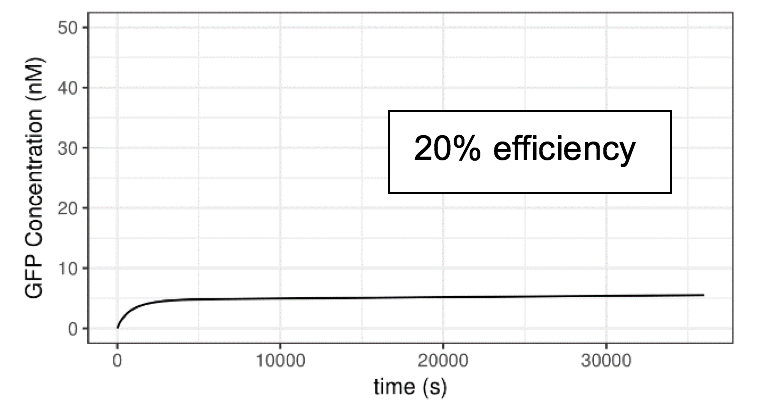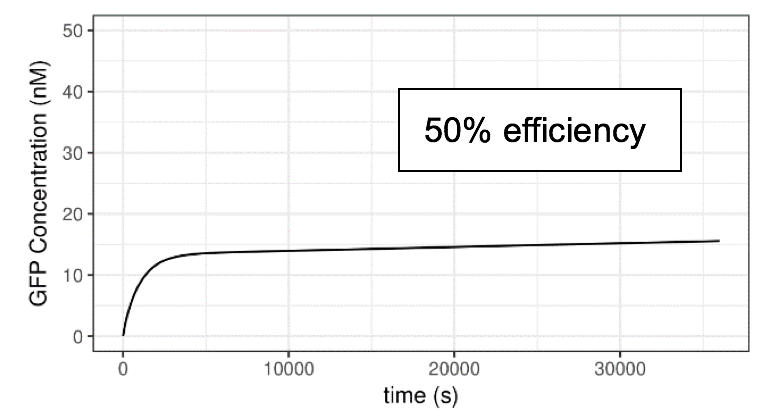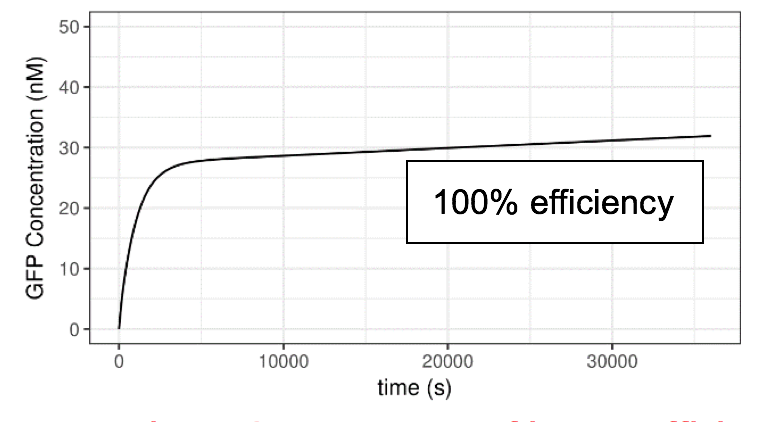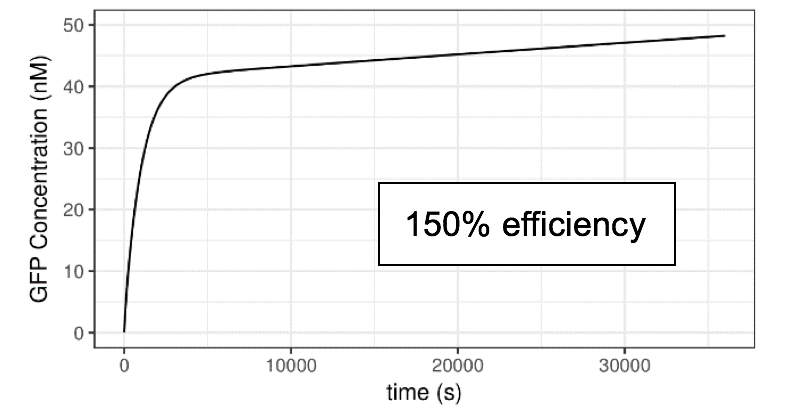Figure 6 Impact of lux pR efficiency (on Neon Plasmid) on the Performance of the System

Clearly, the efficiency of lux pR on the Neon plasmid significantly affects the behavior of the system. An efficient/sensitive lux pR promoter results in a faster increase of GFP concentration. This also accords with our further experimental data.
Tuning the activity of the lux pR on the safety catch promoter also alters the behavior of the system. We set the lux pR on the Neon plasmid as the original one, while remaining AHL concentration as 10-6 M, and then tune the efficiency of lux pR on the safety catch plasmid to produce the plots below.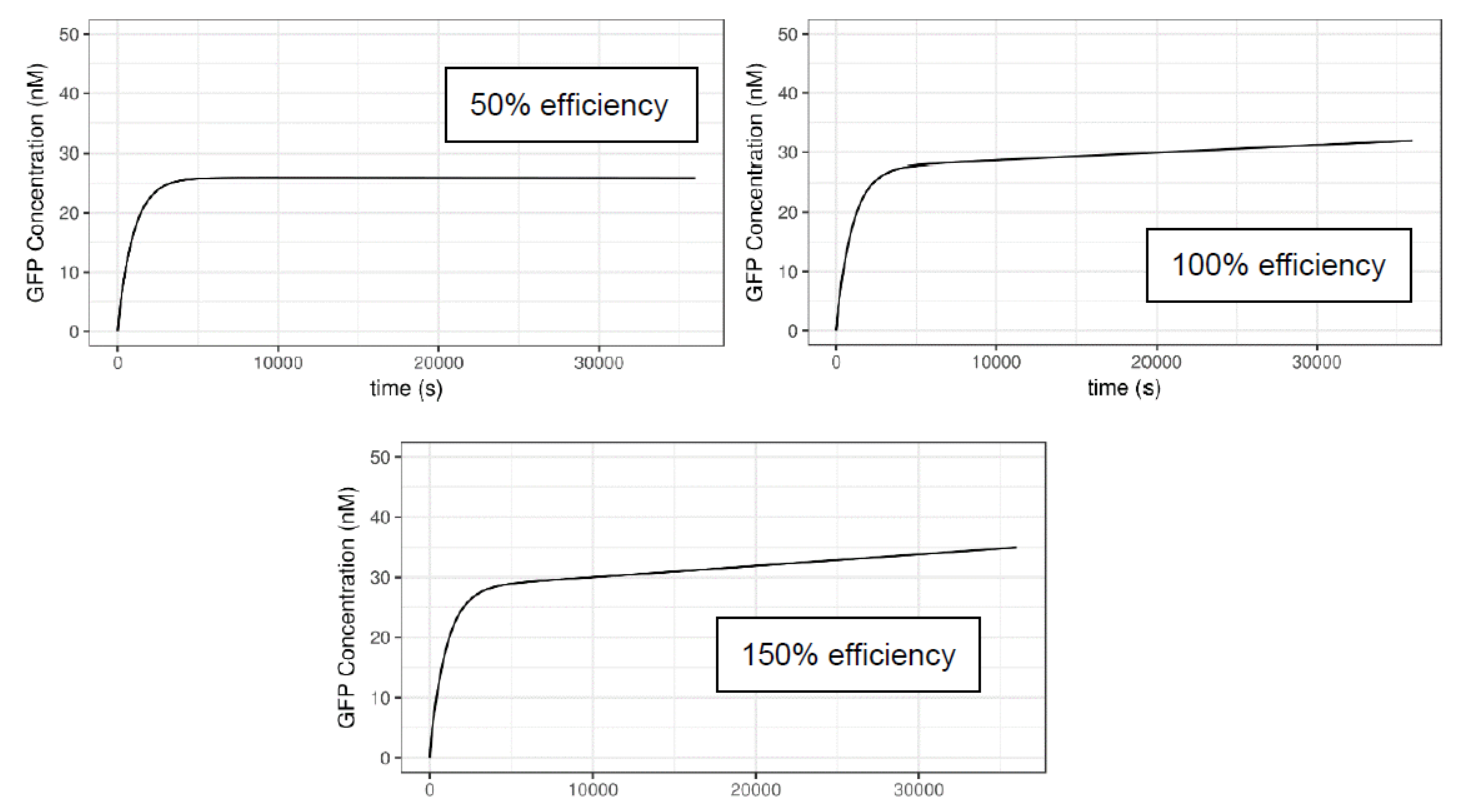Figure 7 Impact of lux pR efficiency (on Safety Catch Plasmid) on the Performance of the System

Obviously, the effect of lux pR promoter on both plasmids can interact can produce a variety of results. A heatmap shows how their interaction alters the productivity of GFP. The color indicates the relative concentration of GFP at “30000 s” time point.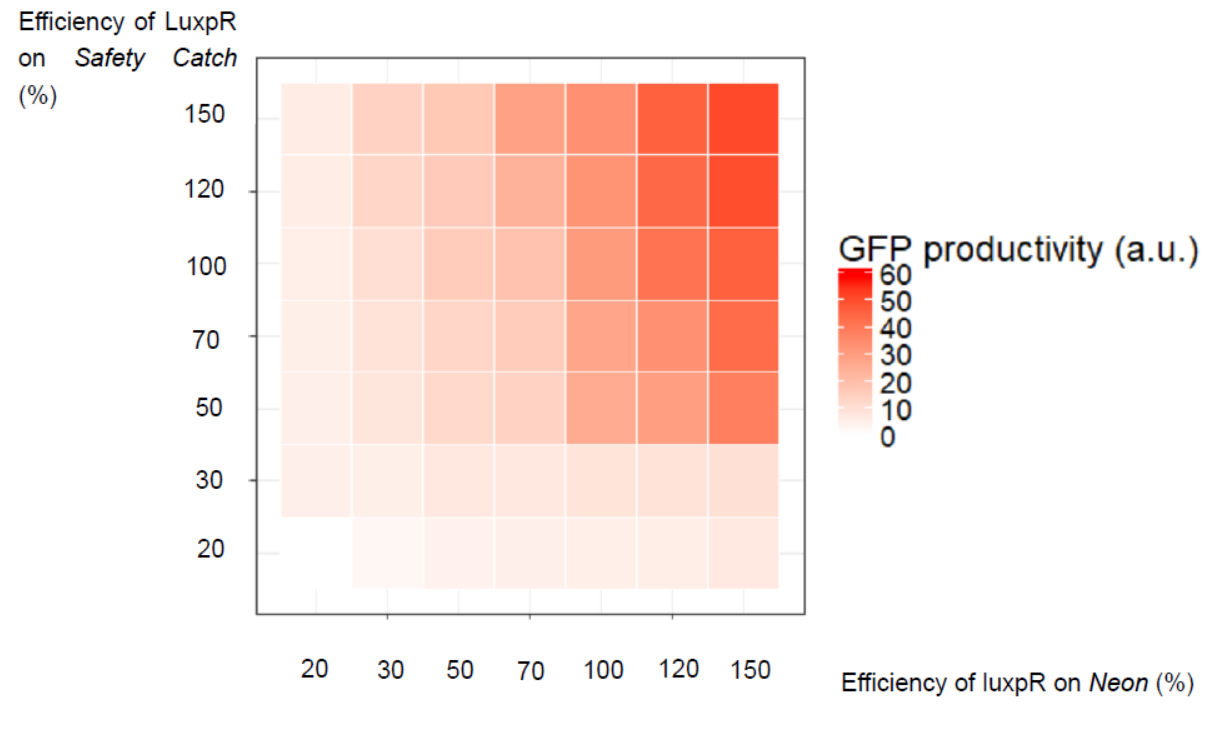Figure 8 Interaction of Effects of lux pR on Two Plasmids

You can interact with the plot down below. Use mouse to drag the slider to tune the lux pR efficiency and observe how this will influence GFP production in the cells!
Summary:
- lux pR efficiency on both plasmids has great impact on the system.
- lux pR efficiency on both plasmids can interact and determine the performance of the system.

3.3 Effect of lux pR leakage on Safety Catch plasmid
The leakage of lux pR refers to the extent of transcription driven by lux pR promoters not bound by AHL-luxR complexes. The leakage of lux pR is anticipated to have impact on the system as well.
The lux pR on Safety Catch plasmid serves as a vital “switch” that determines how easily the system can be activated. If it has a higher leakage level, the lacI expression driven by it should be stronger, thus inhibiting the expression gRNA, making the system more sensitive to AHL’s activation effect. On the other hand, lux pR with strictly low leakage level might result in a system difficult to induce. An optimal level of leakage of lux pR might be a key factor to building an optimal system. Herein, we use our model simulation to validate these thoughts.
As shown below, the AHL concentration is set to be 10-8M, which should not be suffient to induce the system. If we increase the leakage level of lux pR on safety catch, the system becomes active under this condition and GFP is produced readily. It seems that the more “leaky” the promoter is, the more easily can the system be induced.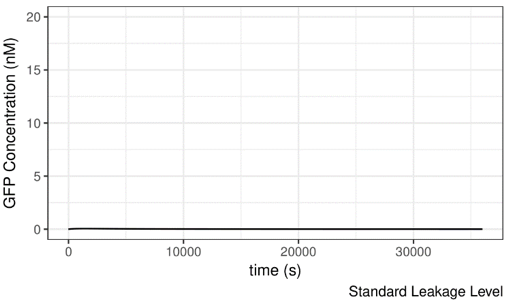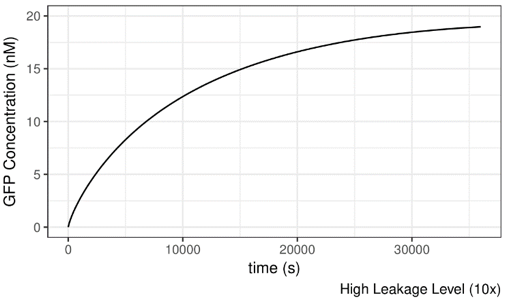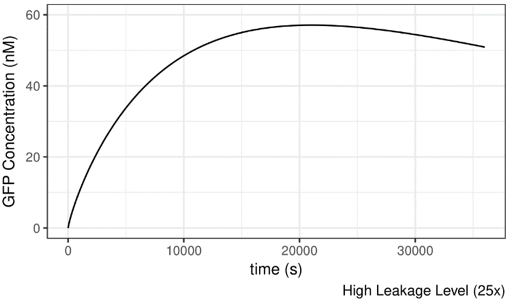Figure 9 Impact of lux pR leakage (on Safety Catch Plasmid) on the Performance of the System

Based on the previous results, both the transcription efficiency and the leakage level of lux pR seems to be crucial to the performance of the system. An optimal combination of transcription efficiency and leakage level is required. Thus, we seek to obtain a better mutant form of lux pR. In the end, we developed lux pR-HS, an improved version of the promoter.
Summary:
- lux pR leakage on Safety Catch plasmid influences the “threshold” of the system.
- Both leakage and transcription efficiency when activated should be taken into consideration when building an optimal promoter.

3.4 Effect of IPTG
IPTG can be added to the system to eliminate the effect of lacI. Addition of IPTG should result in increased production of guide RNA, making the system more difficult to induce. In other words, lacI helps to modulate the threshold of system activation and adjusts the rate of GFP synthesis. To validate this hypothesis, we run simulations of our model.

#### 4.Sensitivity Test and Robustness of the System

4.1 Overview
What is robustness? Robustness means some properties of the system are able to remain the same under perturbations. In our case, if our model is "robust" to some extent, then perturbations of certain "inputs" of the model should cast little effect on the "outputs". As for the "inputs", they can be the initial concentrations of chemical species and biological parameters. As for the outputs, they are the simulated GFP concentrations at given time points.
First of all, we would like to determine whether the model is robust given perturbations in parameter values. theoretically, none of the values of these parameters can be exactly precise, so confirming that minor shifts from ideal values do not cause the result of simulation dramatically is naturally important. Robustness to parameter values is somehow closely related to "sensitivity" and "sensitivity test".... The sensitivities of an ODE system are defined as the derivatives of the solution with respect to the parameters. We choose to perform Global Sensitivity Analysis, which is meant to be used for exploring the sensitivity over a larger domain without calculating derivatives.
A scan of parameters as well as sensitivity test can be done using R, with the package ODEsensitivity. We primarily employ the "Morris Method" for global sensitivity analysis. Morris Method is also known as Morris' OAT Method, where OAT stands for "One At a Time". Namely, when using Morris Method, one parameter value undergoes is changed during each run. Parameters change within a confined parameter space, while a sensitivity measure named "the elementary effect" is calculated during each run.
$$[EE_i=\frac{f(x_1,x_2,...,x_i+\delta ,...,x_k)-y}{\delta}$$

In the test, $\mu^*$, the mean of the elementary effects, is used to measure the influence of a parameter on the output. The larger it is, the more this parameter would influence the model. $\sigma$, the variance, is a measure of non-linearity and/or interaction effects of the parameter.
4.2 Sensitivity Test of the Parameters
What is robustness? Robustness means some properties of the system are able to remain the same under perturbations. In our case, if our model is "robust" Here are some plots showing the elementary effects of several parameters. As expected, $\mu^*$ and $\sigma$ tend to increase over time.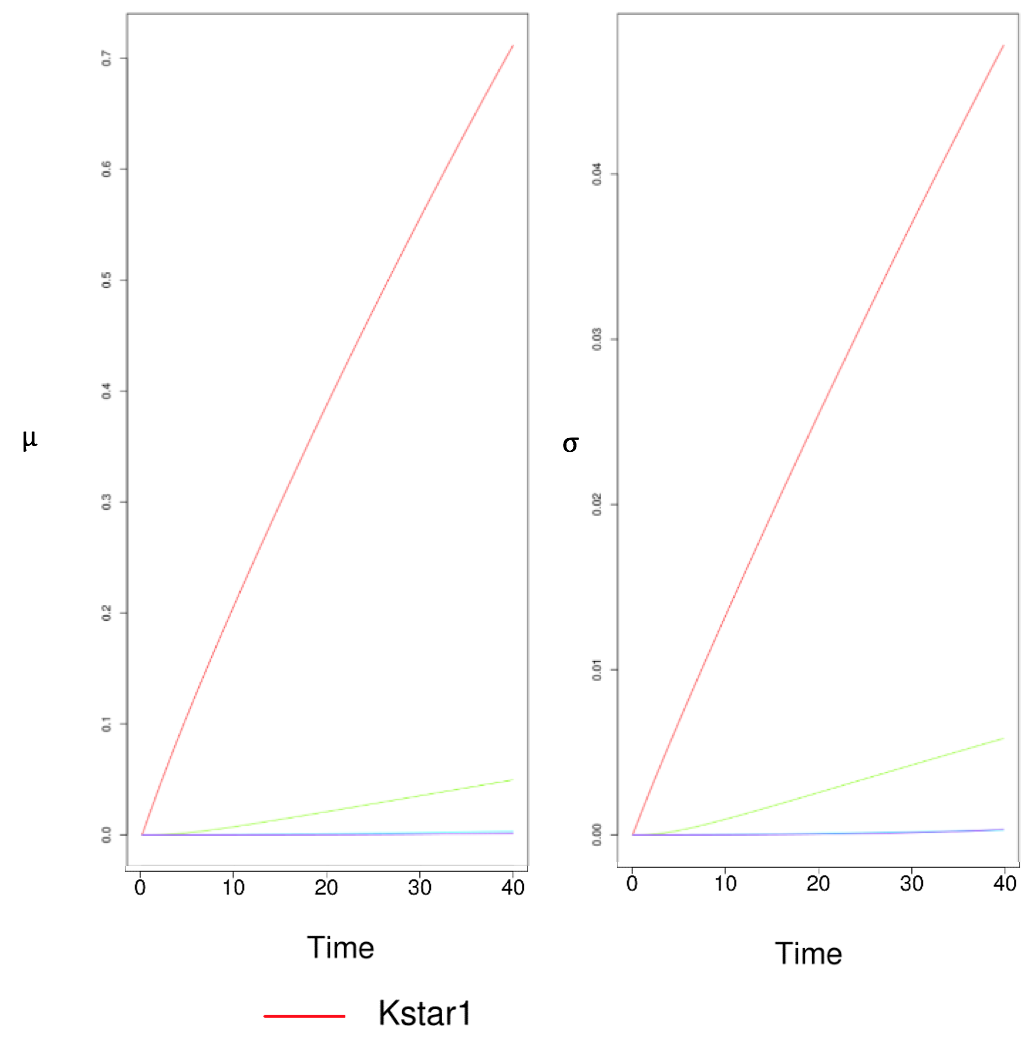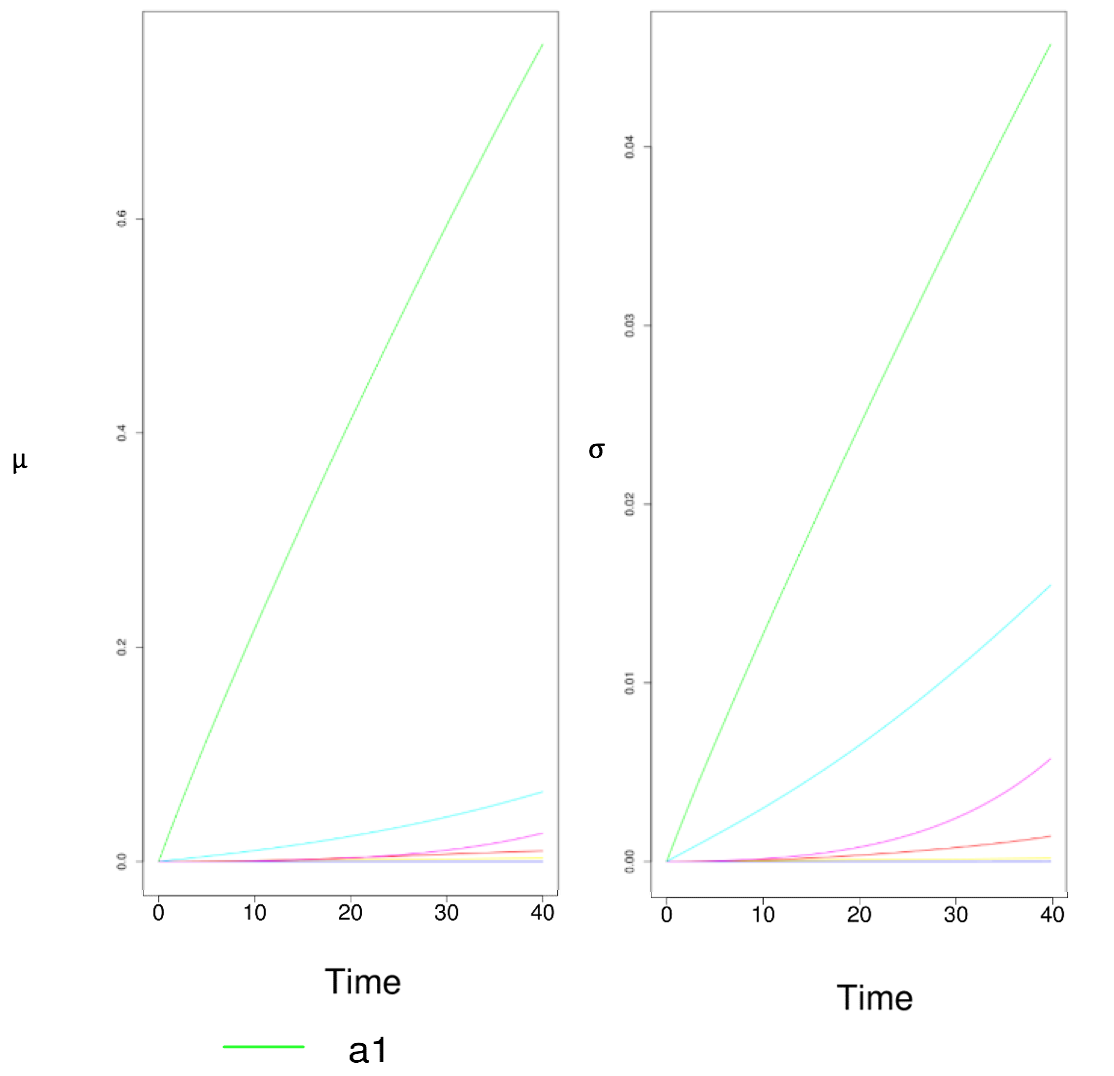Figure 10 Results of Sensitivity Test. Two Parameters Have Especially Large Impacts on the System When Perturbed.

These are just two pieces of snapshots of the results of a series of scans. Apparently, two parameters stand out to be much more sensitive than any other parameters. The lines that represent them are specially highlighted. These two parameters stand for “efficiency of lux pR promoter” and “binding affinity of luxR-AHL to lux pR”. Intriguingly, these two parameters involve lux pR, which is a primary interest in our project. In previous parts, we have already analyzed how lux pR efficiency greatly impacts our system. This accords with the conclusion drawn from the sensitivity test as well.
Following numerous repetitive runs of sensitivity test, we confirmed the only those two parameters addressed above are significantly sensitive to perturbations.
Based on this, we can say that our system is generally robust, as minor changes to the majority of the parameter values does not significantly affect the results. For now, our simulation results can be trusted to be useful.
Summary:
- The system is generally robust and stable according to our sensitivity analysis.
- The parameters related to properties of lux pR are relatively more sensitive to perturbations. This accords with our previous conclusions.

References
 Marc, W., & Javier, B. (2013). Dynamics of the quorum sensing switch: stochastic and non-stationary effects. BMC Systems Biology,7,1(2013-01-16), 7(1), 6-6.
 James, S., Nilsson, P., James, G., Kjelleberg, S., & Fagerström, T. (2000). Luminescence control in the marine bacterium vibrio fischeri: an analysis of the dynamics of lux regulation. Journal of Molecular Biology, 296(4), 1127-1137.
 Samuel E Clamons, Richard M Murray (2017) Modeling Dynamic Transcriptional Circuits with CRISPRi. BioRxiv
 Saeidi, N., Arshath, M., Chang, M. W., & Poh, C. L. (2013). Characterization of a quorum sensing device for synthetic biology design: experimental and modeling validation. Chemical Engineering Science, 103(22), 91-99.
 Huynh, L., & Tagkopoulos, I. (2016). A parts database with consensus parameter estimation for synthetic circuit design. Acs Synthetic Biology, 5(12).